# ode113

Solve nonstiff differential equations — variable order method

## Syntax

[t,y] = ode113(odefun,tspan,y0)
[t,y] = ode113(odefun,tspan,y0,options)
[t,y,te,ye,ie] = ode113(odefun,tspan,y0,options)
sol = ode113(___)

## Description

example

[t,y] = ode113(odefun,tspan,y0), where tspan = [t0 tf], integrates the system of differential equations $y\text{'}=f\left(t,y\right)$ from t0 to tf with initial conditions y0. Each row in the solution array y corresponds to a value returned in column vector t.All MATLAB® ODE solvers can solve systems of equations of the form $y\text{'}=f\left(t,y\right)$, or problems that involve a mass matrix, $M\left(t,y\right)y\text{'}=f\left(t,y\right)$. The solvers all use similar syntaxes. The ode23s solver only can solve problems with a mass matrix if the mass matrix is constant. ode15s and ode23t can solve problems with a mass matrix that is singular, known as differential-algebraic equations (DAEs). Specify the mass matrix using the Mass option of odeset.

example

[t,y] = ode113(odefun,tspan,y0,options) also uses the integration settings defined by options, which is an argument created using the odeset function. For example, use the AbsTol and RelTol options to specify absolute and relative error tolerances, or the Mass option to provide a mass matrix.
[t,y,te,ye,ie] = ode113(odefun,tspan,y0,options) additionally finds where functions of (t,y), called event functions, are zero. In the output, te is the time of the event, ye is the solution at the time of the event, and ie is the index of the triggered event.For each event function, specify whether the integration is to terminate at a zero and whether the direction of the zero crossing matters. Do this by setting the 'Events' property to a function, such as myEventFcn or @myEventFcn, and creating a corresponding function: [value,isterminal,direction] = myEventFcn(t,y). For more information, see ODE Event Location.
sol = ode113(___) returns a structure that you can use with deval to evaluate the solution at any point on the interval [t0 tf]. You can use any of the input argument combinations in previous syntaxes.

## Examples

collapse all

Simple ODEs that have a single solution component can be specified as an anonymous function in the call to the solver. The anonymous function must accept two inputs (t,y), even if one of the inputs is not used in the function.

Solve the ODE

${y}^{\prime }=2t.$

Specify a time interval of [0 5] and the initial condition y0 = 0.

tspan = [0 5]; y0 = 0; [t,y] = ode113(@(t,y) 2*t, tspan, y0);

Plot the solution.

plot(t,y,'-o')The van der Pol equation is a second-order ODEwhere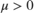is a scalar parameter. Rewrite this equation as a system of first-order ODEs by making the substitution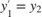. The resulting system of first-order ODEs is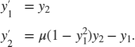The function file vdp1.m represents the van der Pol equation using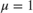. The variablesand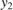are the entries y(1) and y(2) of a two-element vector dydt.

function dydt = vdp1(t,y) %VDP1 Evaluate the van der Pol ODEs for mu = 1 % % See also ODE113, ODE23, ODE45. % Jacek Kierzenka and Lawrence F. Shampine % Copyright 1984-2014 The MathWorks, Inc. dydt = [y(2); (1-y(1)^2)*y(2)-y(1)]; 

Solve the ODE using the ode113 function on the time interval [0 20] with initial values [2 0]. The resulting output is a column vector of time points t and a solution array y. Each row in y corresponds to a time returned in the corresponding row of t. The first column of y corresponds to, and the second column corresponds to.

[t,y] = ode113(@vdp1,[0 20],[2; 0]); 

Plot the solutions forandagainst t.

plot(t,y(:,1),'-o',t,y(:,2),'-o') title('Solution of van der Pol Equation (\mu = 1) with ODE113'); xlabel('Time t'); ylabel('Solution y'); legend('y_1','y_2')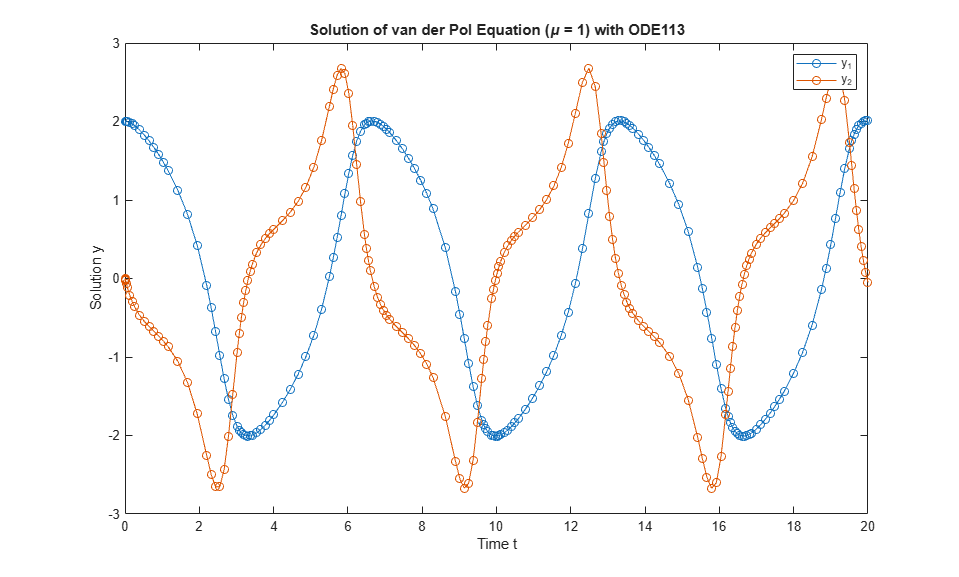ode113 only works with functions that use two input arguments, t and y. However, you can pass in extra parameters by defining them outside the function and passing them in when you specify the function handle.

Solve the ODERewriting the equation as a first-order system yields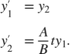odefcn.m represents this system of equations as a function that accepts four input arguments: t, y, A, and B.

function dydt = odefcn(t,y,A,B) dydt = zeros(2,1); dydt(1) = y(2); dydt(2) = (A/B)*t.*y(1); 

Solve the ODE using ode113. Specify the function handle such that it passes in the predefined values for A and B to odefcn.

A = 1; B = 2; tspan = [0 5]; y0 = [0 0.01]; [t,y] = ode113(@(t,y) odefcn(t,y,A,B), tspan, y0); 

Plot the results.

plot(t,y(:,1),'-o',t,y(:,2),'-.')Compared to ode45, the ode113, ode78, and ode89 solvers are better at solving problems with stringent error tolerances. A common situation where ode113 excels is in orbital dynamics problems, where the solution curve is smooth and requires high accuracy.

The two-body problem considers two interacting masses m1 and m2 orbiting in a common plane. In this example, one of the masses is significantly larger than the other. With the heavy body at the origin, the equations of motion arewhereTo solve the problem, first convert to a system of four first-order ODEs using the substitutionsThe substitutions produce the first-order systemThe function twobodyode codes the system of equations for the two-body problem.

function dy = twobodyode(t,y) % Two body problem with one mass much larger than the other. r = sqrt(y(1)^2 + y(3)^2); dy = [y(2); -y(1)/r^3; y(4); -y(3)/r^3]; 

Save twobodyode.m in your working directory, then solve the ODE using ode113. Specify stringent error tolerances of 1e-13 for RelTol and 1e-14 for AbsTol.

opts = odeset('Reltol',1e-13,'AbsTol',1e-14,'Stats','on'); tspan = [0 10*pi]; y0 = [2 0 0 0.5]; [t,y] = ode113(@twobodyode, tspan, y0, opts); plot(t,y) legend('x','x''','y','y''','Location','SouthEast') title('Position and Velocity Components') 
924 successful steps 4 failed attempts 1853 function evaluationsfigure plot(y(:,1),y(:,3),'-o',0,0,'ro') axis equal title('Orbit of Smaller Mass')Compared to ode45, the ode113 solver is able to obtain the solution faster and with fewer function evaluations.

## Input Arguments

collapse all

Functions to solve, specified as a function handle that defines the functions to be integrated.

The function dydt = odefun(t,y), for a scalar t and a column vector y, must return a column vector dydt of data type single or double that corresponds to $f\left(t,y\right)$. odefun must accept both input arguments t and y, even if one of the arguments is not used in the function.

For example, to solve $y\text{'}=5y-3$, use the function:

function dydt = odefun(t,y) dydt = 5*y-3; end

For a system of equations, the output of odefun is a vector. Each element in the vector is the computed value of the right side of one equation. For example, consider the system of two equations

$\begin{array}{l}y{\text{'}}_{1}={y}_{1}+2{y}_{2}\\ y{\text{'}}_{2}=3{y}_{1}+2{y}_{2}\end{array}$

A function that calculates the value of the right side of each equation at each time step is

function dydt = odefun(t,y) dydt = zeros(2,1); dydt(1) = y(1)+2*y(2); dydt(2) = 3*y(1)+2*y(2); end

For information on how to provide additional parameters to the function odefun, see Parameterizing Functions.

Example: @myFcn

Data Types: function_handle

Interval of integration, specified as a vector. At a minimum, tspan must be a two-element vector [t0 tf] specifying the initial and final times. To obtain solutions at specific times between t0 and tf, use a longer vector of the form [t0,t1,t2,...,tf]. The elements in tspan must be all increasing or all decreasing.

The solver imposes the initial conditions given by y0 at the initial time tspan(1), and then integrates from tspan(1) to tspan(end):

• If tspan has two elements [t0 tf], then the solver returns the solution evaluated at each internal integration step within the interval.

• If tspan has more than two elements [t0,t1,t2,...,tf], then the solver returns the solution evaluated at the given points. However, the solver does not step precisely to each point specified in tspan. Instead, the solver uses its own internal steps to compute the solution, and then evaluates the solution at the requested points in tspan. The solutions produced at the specified points are of the same order of accuracy as the solutions computed at each internal step.

Specifying several intermediate points has little effect on the efficiency of computation, but can affect memory management for large systems.

The values of tspan are used by the solver to calculate suitable values for InitialStep and MaxStep:

• If tspan contains several intermediate points [t0,t1,t2,...,tf], then the specified points give an indication of the scale for the problem, which can affect the value of InitialStep used by the solver. Therefore, the solution obtained by the solver might be different depending on whether you specify tspan as a two-element vector or as a vector with intermediate points.

• The initial and final values in tspan are used to calculate the maximum step size MaxStep. Therefore, changing the initial or final values in tspan can cause the solver to use a different step sequence, which might change the solution.

Example: [1 10]

Example: [1 3 5 7 9 10]

Data Types: single | double

Initial conditions, specified as a vector. y0 must be the same length as the vector output of odefun, so that y0 contains an initial condition for each equation defined in odefun.

Data Types: single | double

Option structure, specified as a structure array. Use the odeset function to create or modify the options structure. See Summary of ODE Options for a list of the options compatible with each solver.

Example: options = odeset('RelTol',1e-5,'Stats','on','OutputFcn',@odeplot) specifies a relative error tolerance of 1e-5, turns on the display of solver statistics, and specifies the output function @odeplot to plot the solution as it is computed.

Data Types: struct

## Output Arguments

collapse all

Evaluation points, returned as a column vector.

• If tspan contains two elements [t0 tf], then t contains the internal evaluation points used to perform the integration.

• If tspan contains more than two elements, then t is the same as tspan.

Solutions, returned as an array. Each row in y corresponds to the solution at the value returned in the corresponding row of t.

Time of events, returned as a column vector. The event times in te correspond to the solutions returned in ye, and ie specifies which event occurred.

Solution at time of events, returned as an array. The event times in te correspond to the solutions returned in ye, and ie specifies which event occurred.

Index of triggered event function, returned as a column vector. The event times in te correspond to the solutions returned in ye, and ie specifies which event occurred.

Structure for evaluation, returned as a structure array. Use this structure with the deval function to evaluate the solution at any point in the interval [t0 tf]. The sol structure array always includes these fields:

Structure FieldDescription

sol.x

Row vector of the steps chosen by the solver.

sol.y

Solutions. Each column sol.y(:,i) contains the solution at time sol.x(i).

sol.solver

Solver name.

Additionally, if you specify the Events option of odeset and events are detected, then sol also includes these fields:

Structure FieldDescription

sol.xe

Points when events occurred. sol.xe(end) contains the exact point of a terminal event, if any.

sol.ye

Solutions that correspond to events in sol.xe.

sol.ie

Indices into the vector returned by the function specified in the Events option. The values indicate which event the solver detected.

## Algorithms

ode113 is a variable-step, variable-order (VSVO) Adams-Bashforth-Moulton PECE solver of orders 1 to 13. The highest order used appears to be 12, however, a formula of order 13 is used to form the error estimate and the function does local extrapolation to advance the integration at order 13.

ode113 may be more efficient than ode45 at stringent tolerances or if the ODE function is particularly expensive to evaluate. ode113 is a multistep solver — it normally needs the solutions at several preceding time points to compute the current solution , .

 Shampine, L. F. and M. K. Gordon, Computer Solution of Ordinary Differential Equations: the Initial Value Problem, W. H. Freeman, San Francisco, 1975.

 Shampine, L. F. and M. W. Reichelt, “The MATLAB ODE Suite,” SIAM Journal on Scientific Computing, Vol. 18, 1997, pp. 1–22.